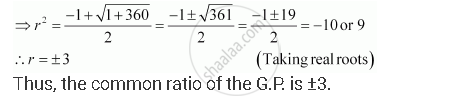CBSE (Arts) Class 11CBSE
Share
Notifications

View all notifications
Books Shortlist
Your shortlist is empty

# The First Term of a G.P. is 1. the Sum of the Third Term and Fifth Term is 90. Find the Common Ratio of Geometric Progression - CBSE (Arts) Class 11 - Mathematics

Login
Create free account

Forgot password?
ConceptGeometric Progression (G. P.)

#### Question

The first term of a G.P. is 1. The sum of the third term and fifth term is 90. Find the common ratio of G.P.

#### Solution

Let a and r be the first term and the common ratio of the G.P. respectively.

∴ a = 1

a3 = ar2 = r2

a5 = ar4 = r4

∴ r2 + r4 = 90

⇒ r4 + r2 – 90 = 0Is there an error in this question or solution?

#### APPEARS IN

NCERT Solution for Mathematics Textbook for Class 11 (2018 to Current)
Chapter 9: Sequences and Series
Q: 9 | Page no. 199
Solution The First Term of a G.P. is 1. the Sum of the Third Term and Fifth Term is 90. Find the Common Ratio of Geometric Progression Concept: Geometric Progression (G. P.).
S